# 700 How many milliliters of a 0.10 M Cuso, solution contain 1.25 gCuSO,?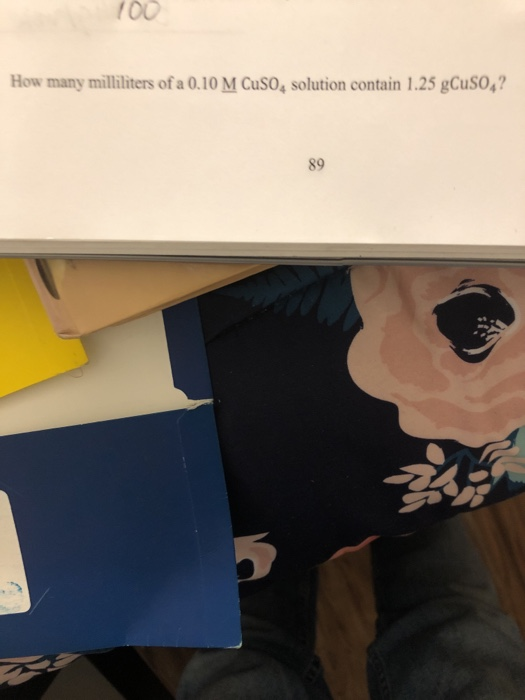700 How many milliliters of a 0.10 M Cuso, solution contain 1.25 gCuSO,?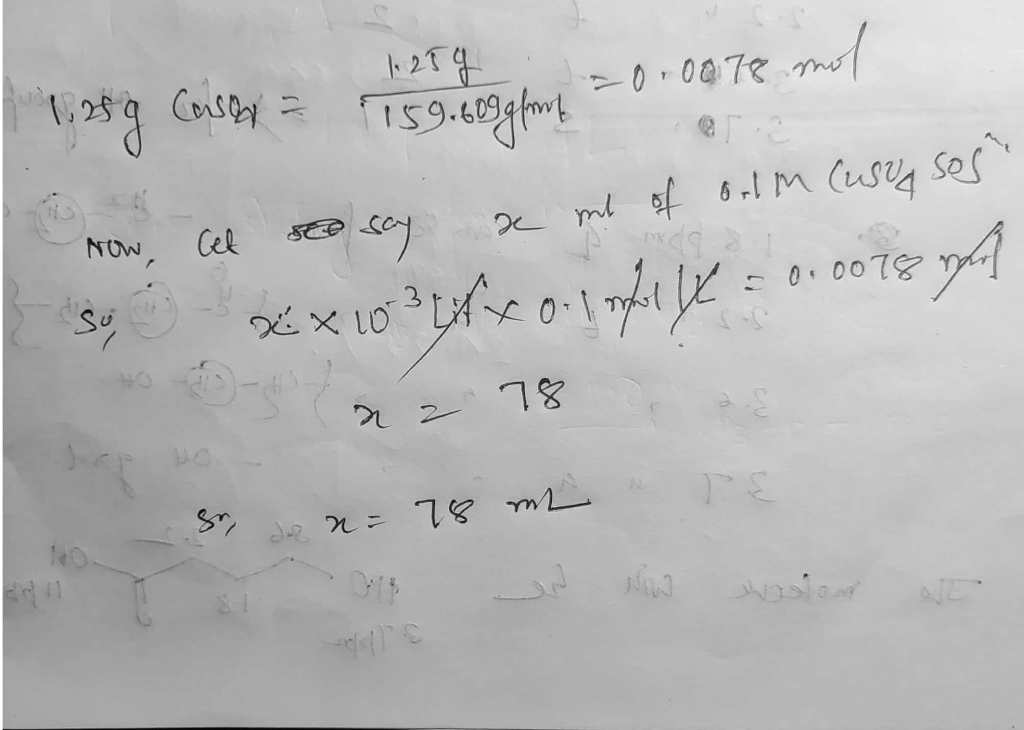#### Earn Coin

Coins can be redeemed for fabulous gifts.

Similar Homework Help Questions
• ### how many milliliters of 20% HBr solution contain 4 grams of HBr? 2. How many milliliters...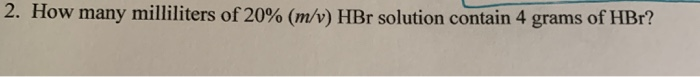how many milliliters of 20% HBr solution contain 4 grams of HBr? 2. How many milliliters of 20% (m/v) HBr solution contain 4 grams of HBr?

• ### How many milliliters of a 0.222 M LiF solution contain 0.444 moles of LiF?

How many milliliters of a 0.222 M LiF solution contain 0.444 moles of LiF?

• ### How many milliliters of 0.10 M sodium sulfide solution are required to precipitate all the nickel,...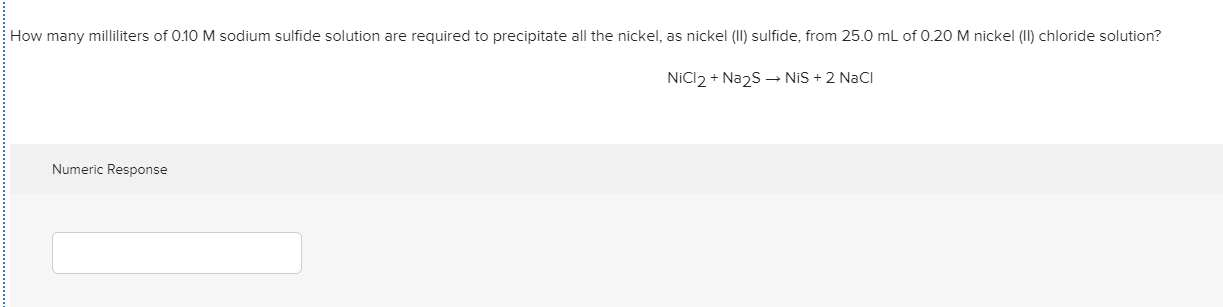How many milliliters of 0.10 M sodium sulfide solution are required to precipitate all the nickel, as nickel (II) sulfide, from 25.0 mL of 0.20 M nickel (II) chloride solution? NiCl2 + Na2S - NIS + 2 Naci Numeric Response

• ### 5. How many milliliters (mL) of a 3.277 M calcium nitrate solution would contain 76.50 grams...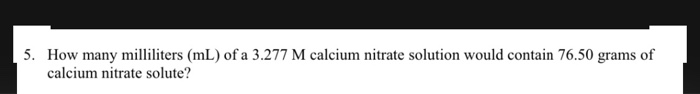5. How many milliliters (mL) of a 3.277 M calcium nitrate solution would contain 76.50 grams of calcium nitrate solute?

• ### Be sure to answer all parts. How many milliliters of a 0.24 M sucrose solution contain...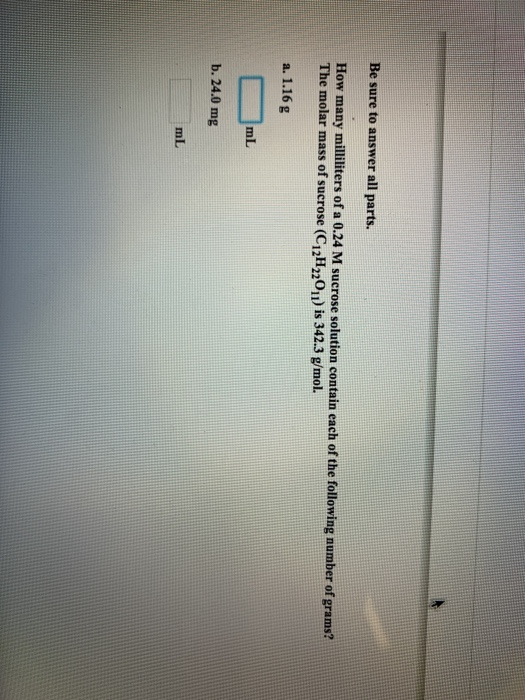Be sure to answer all parts. How many milliliters of a 0.24 M sucrose solution contain each of the following number of grams? The molar mass of sucrose (C12H22011) is 342.3 g/mol. a. 1.16 g ml b. 24.0 mg

• ### Be sure to answer all parts. How many milliliters of a 0.42 M sucrose solution contain...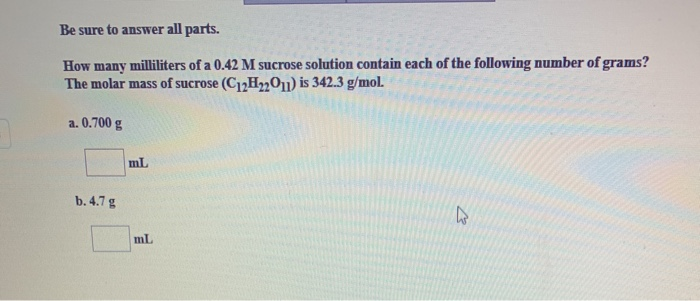Be sure to answer all parts. How many milliliters of a 0.42 M sucrose solution contain each of the following number of grams? The molar mass of sucrose (C12H2011) is 342.3 g/mol a. 0.700 g ml b.4.7 g

• ### How many milliliters of 0.10 M HCl must be added to 52.0 mL of 0.40 M HCl to give a final solution that has a molarity of 0.25 M

How many milliliters of 0.10 M HCl must be added to 52.0 mL of 0.40 M HCl to give a final solution that has a molarity of 0.25 M?

• ### Question 13: How many milliliters of a 0.45 M solution contain 15.0 g of BaCl2? Express...

Question 13: How many milliliters of a 0.45 M solution contain 15.0 g of BaCl2? Express your answer using two significant figures Question 14: A bottle of 12.0 M hydrochloric acid has only 35.4 mL left in it. A)What will the HCl concentration be if the solution is diluted to 270.0 mL ?

• ### How many milliliters of a 0.450 M LiI solution contain 0.600 moles of LiI? Group of...

How many milliliters of a 0.450 M LiI solution contain 0.600 moles of LiI? Group of answer choices 0.885 x 103 mL 0.326 x 103 mL 0.750 x 103 mL 1.33 x 103 mL 0.543 x 103 mL

• ### 3. How many milliliters of a 20% magnesium sulfate solution will contain 40 grams of the...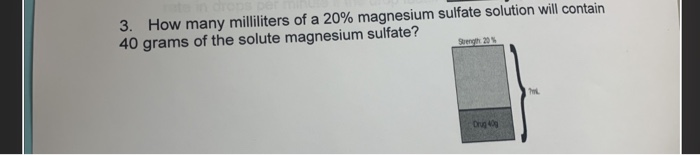3. How many milliliters of a 20% magnesium sulfate solution will contain 40 grams of the solute magnesium sulfate?